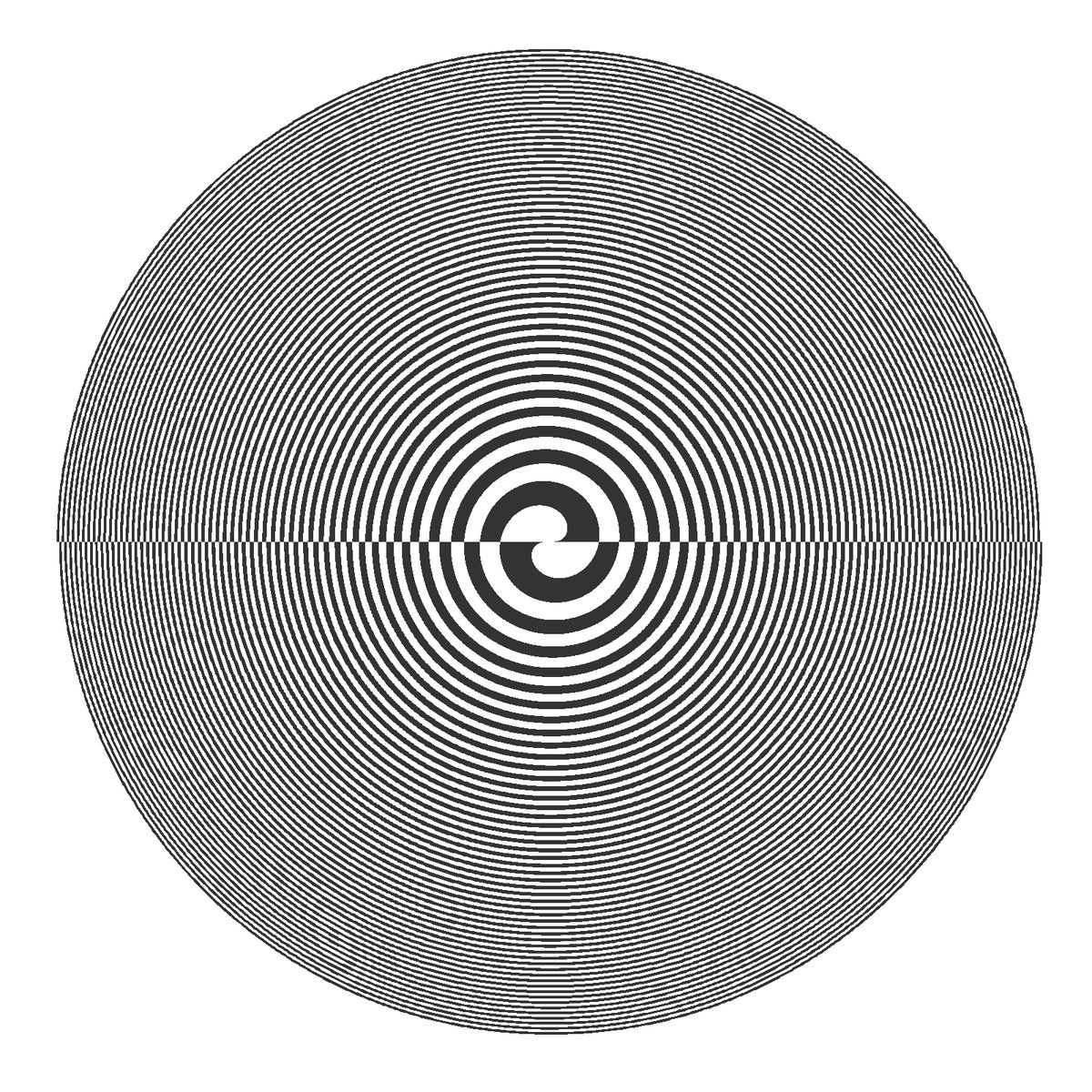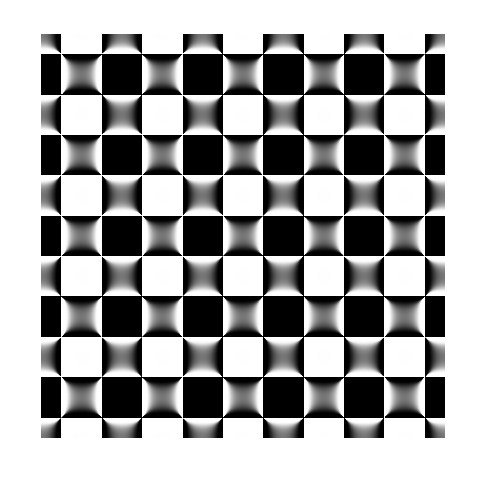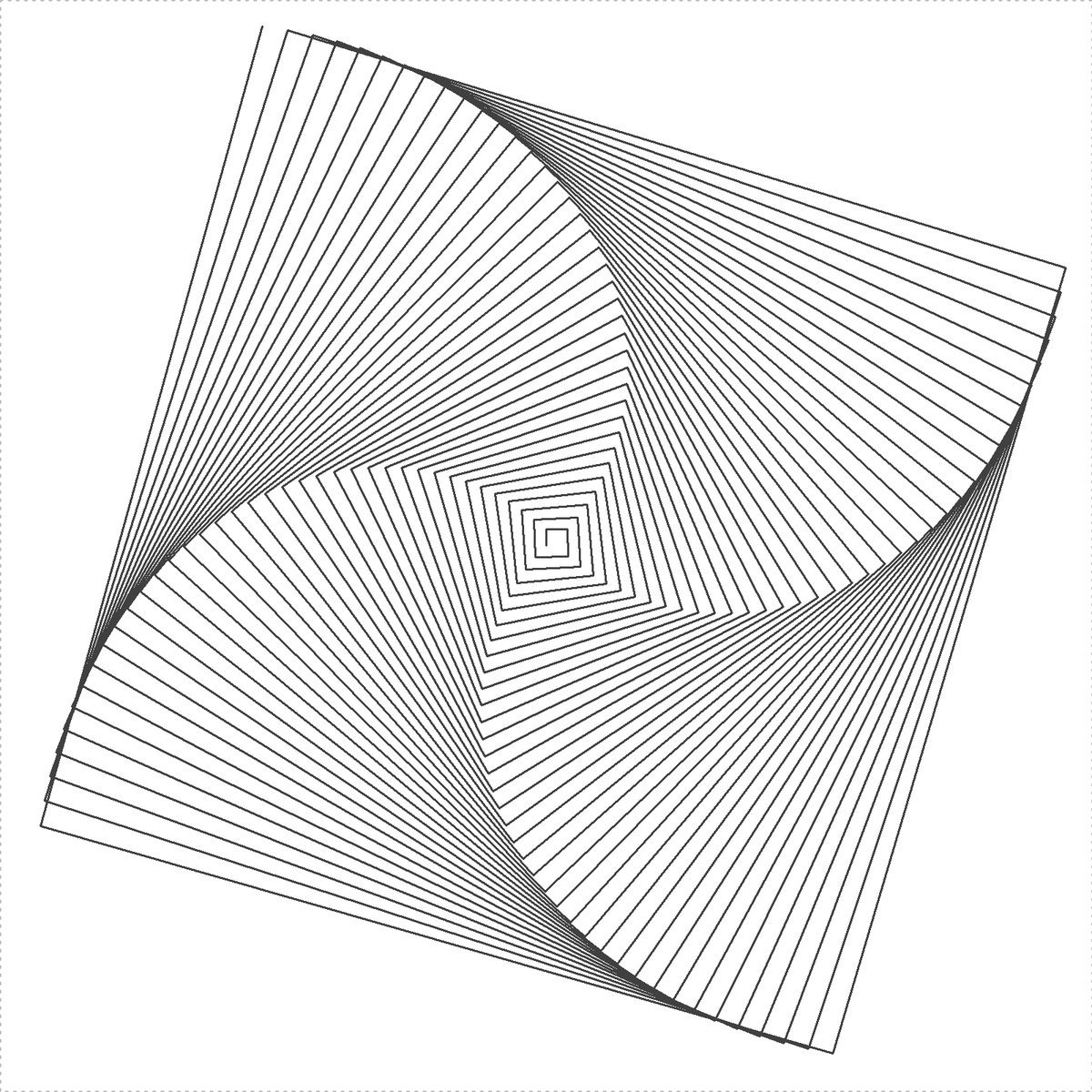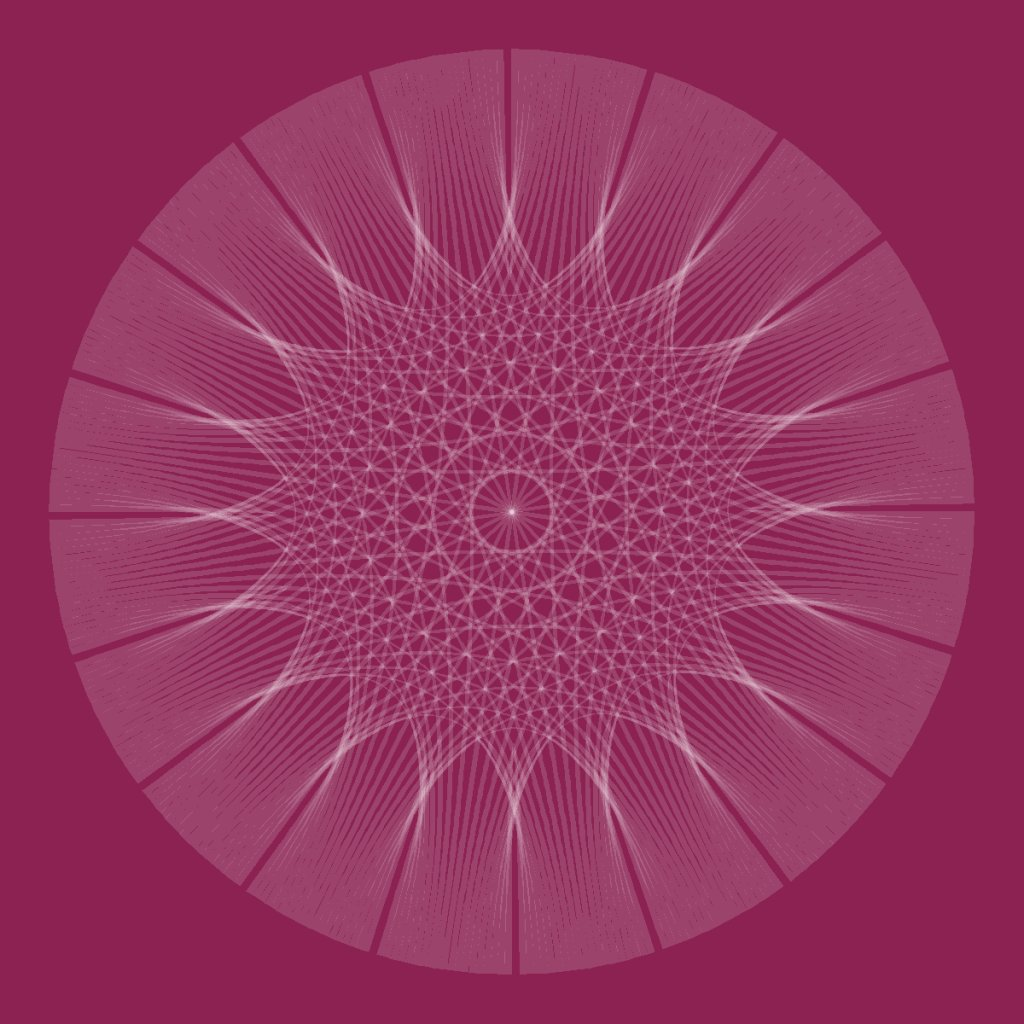Tiny Art in Less Than 280 Characters

Now that Twitter allows 280 characters, the code of some drawings I have made can fit in a tweet. In this post I have compiled a few of them.

The first one is a cardioid inspired in string art (more info here):

library(ggplot2)
n=300
t1=1:n
t0=seq(3,2*n+1,2)%%n
t2=t0+(t0==0)*n
df=data.frame(x=cos((t1-1)*2*pi/n),
y=sin((t1-1)*2*pi/n),
x2=cos((t2-1)*2*pi/n),
y2=sin((t2-1)*2*pi/n))
ggplot(df,aes(x,y,xend=x2,yend=y2)) +
geom_segment(alpha=.1)+theme_void()library(ggplot2)
library(dplyr)
t=seq(from=0, to=100*pi, length.out=500*100)
data.frame(x= t^(1/2)*cos(t), y= t^(1/2)*sin(t))%>%
rbind(-.)%>%ggplot(aes(x, y))+geom_polygon()+theme_void()library(dplyr)
library(ggplot2)
library(pracma)
seq(-5*pi,5*pi,by=.1)%>%expand.grid(x=., y=.)%>%
ggplot(aes(x=x, y=y, fill=erf(sec(x)-sec(y))))+geom_tile()+
theme_void()+theme(legend.position="none")A x-y scatter plot of a trigonometric function on R2 (more info here):

library(dplyr)
library(ggplot2)
seq(from=-10, to=10, by = 0.05) %>%
expand.grid(x=., y=.) %>%
ggplot(aes(x=(x+pi*sin(y)), y=(y+pi*sin(x)))) +
geom_point(alpha=.1, shape=20, size=1, color="black")+
theme_void()library(TurtleGraphics)
turtle_init()
turtle_col("gray25")
turtle_do({
for (i in 1:150) {
turtle_forward(dist=1+0.5*i)
turtle_right(angle=89.5)}
})
turtle_hide()t=seq(1, 100, by=.001)
plot(exp(-0.006*t)*sin(t*3.019+2.677)+
exp(-0.001*t)*sin(t*2.959+2.719),
exp(-0.009*t)*sin(t*2.964+0.229)+
exp(-0.008*t)*sin(t*2.984+1.284),
type="l", axes=FALSE, xlab="", ylab="")library(circlize)
chordDiagram(matrix(1, 20, 20), symmetric = TRUE,
col="black", transparency = 0.85, annotationTrack = NULL)Most of them are made with ggplot2 package. I love R and the sense of wonder of how just one or two lines of code can create beautiful and unexpected patterns.

I recently did this project for DataCamp to show how easy is to do art with R and ggplot. Starting from a extremely simple plot, and following a well guided path, you can end making beautiful images like this one:Furthermore, you can learn also ggplot2 while you do art.

I have done the project together with Rasmus Bååth, instructor at DataCamp and the perfect mate to work with. He is looking for people to build more projects so if you are interested, here you can find more information. Do not hesitate to ask him for details.

All the best for 2018.

Merry Christmas.